Courses

Linked lists Notes | Study GATE Computer Science Engineering(CSE) 2022 Mock Test Series - Computer Science Engineering (CSE)

Computer Science Engineering (CSE): Linked lists Notes | Study GATE Computer Science Engineering(CSE) 2022 Mock Test Series - Computer Science Engineering (CSE)

The document Linked lists Notes | Study GATE Computer Science Engineering(CSE) 2022 Mock Test Series - Computer Science Engineering (CSE) is a part of the Computer Science Engineering (CSE) Course GATE Computer Science Engineering(CSE) 2022 Mock Test Series.
All you need of Computer Science Engineering (CSE) at this link: Computer Science Engineering (CSE)

Like arrays, Linked List is a linear data structure. Unlike arrays, linked list elements are not stored at contiguous location; the elements are linked using pointers.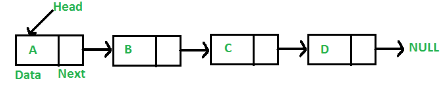Arrays can be used to store linear data of similar types, but arrays have following limitations.
1) The size of the arrays is fixed: So we must know the upper limit on the number of elements in advance. Also, generally, the allocated memory is equal to the upper limit irrespective of the usage.
2) Inserting a new element in an array of elements is expensive, because room has to be created for the new elements and to create room existing elements have to shifted.

For example, in a system if we maintain a sorted list of IDs in an array id[].

id[] = [1000, 1010, 1050, 2000, 2040].

And if we want to insert a new ID 1005, then to maintain the sorted order, we have to move all the elements after 1000 (excluding 1000).
Deletion is also expensive with arrays until unless some special techniques are used. For example, to delete 1010 in id[], everything after 1010 has to be moved.

1) Dynamic size
2) Ease of insertion/deletion

Drawbacks:

1) Random access is not allowed. We have to access elements sequentially starting from the first node. So we cannot do binary search with linked lists.
2) Extra memory space for a pointer is required with each element of the list.

Representation in C:

A linked list is represented by a pointer to the first node of the linked list. The first node is called head. If the linked list is empty, then value of head is NULL.
Each node in a list consists of at least two parts:
1) data
2) pointer to the next node
In C, we can represent a node using structures. Below is an example of a linked list node with an integer data.
In Java, LinkedList can be represented as a class and a Node as a separate class. The LinkedList class contains a reference of Node class type.

struct node
{
int data;
struct node *next;
};

First Simple Linked List in C Let us create a simple linked list with 3 nodes.

// A simple C program to introduce a linked list
#include<stdio.h>
#include<stdlib.h>

struct node
{
int data;
struct node *next;
};

// Program to create a simple linked list with 3 nodes
int main()
{
struct node* second = NULL;
struct node* third = NULL;

// allocate 3 nodes in the heap
second = (struct node*)malloc(sizeof(struct node));
third = (struct node*)malloc(sizeof(struct node));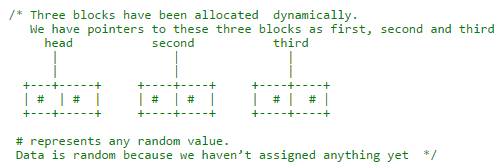head->data = 1; //assign data in first node

/* data has been assigned to data part of first block (block
pointed by head).  And next pointer of first block points to
second.  So they both are linked.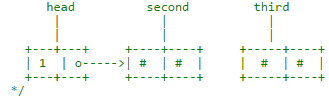second->data = 2; //assign data to second node
second->next = third; // Link second node with the third node

/* data has been assigned to data part of second block (block pointed by
second). And next pointer of the second block points to third block.
So all three blocks are linked.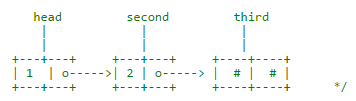third->data = 3; //assign data to third node
third->next = NULL;

/* data has been assigned to data part of third block (block pointed
by third). And next pointer of the third block is made NULL to indicate
that the linked list is terminated here.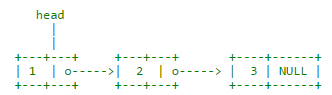Note that only head is sufficient to represent the whole list.  We can
traverse the complete list by following next pointers.    */

return 0;
}

In the previous program, we have created a simple linked list with three nodes. Let us traverse the created list and print the data of each node. For traversal, let us write a general purpose function printList() that prints any given list.

// A simple C program for traversal of a linked list
#include<stdio.h>
#include<stdlib.h>

struct node
{
int data;
struct node *next;
};

// This function prints contents of linked list starting from
// the given node
void printList(struct node *n)
{
while (n != NULL)
{
printf(" %d ", n->data);
n = n->next;
}
}

int main()
{
struct node* second = NULL;
struct node* third = NULL;

// allocate 3 nodes in the heap
second = (struct node*)malloc(sizeof(struct node));
third  = (struct node*)malloc(sizeof(struct node));

head->data = 1; //assign data in first node

second->data = 2; //assign data to second node
second->next = third;

third->data = 3; //assign data to third node
third->next = NULL;

return 0;
}

Doubly Linked List | (Introduction and Insertion)

We strongly recommend to refer following post as a prerequisite of this post.

Inserting a node in Singly Linked List

Doubly Linked List (DLL) contains an extra pointer, typically called previous pointer, together with next pointer and data which are there in singly linked list.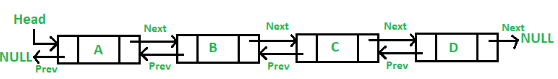Following is representation of a DLL node in C language.
/* Node of a doubly linked list */
struct node
{
int data;
struct node *next; // Pointer to next node in DLL
struct node *prev; // Pointer to previous node in DLL
};

1) A DLL can be traversed in both forward and backward direction.
2) The delete operation in DLL is more efficient if pointer to the node to be deleted is given.
In singly linked list, to delete a node, pointer to the previous node is needed. To get this previous node, sometimes the list is traversed. In DLL, we can get the previous node using previous pointer.

1) Every node of DLL Require extra space for an previous pointer. It is possible to implement DLL with single pointer though.
2) All operations require an extra pointer previous to be maintained. For example, in insertion, we need to modify previous pointers together with next pointers. For example in following functions for insertions at different positions, we need 1 or 2 extra steps to set previous pointer.

Insertion

A node can be added in four ways
1) At the front of the DLL
2) After a given node.
3) At the end of the DLL
4) Before a given node.

1) Add a node at the front: (A 5 steps process)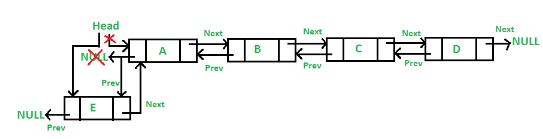Following are the 5 steps to add node at the front.

/* Given a reference (pointer to pointer) to the head of a list
and an int, inserts a new node on the front of the list. */
void push(struct node** head_ref, int new_data)
{
/* 1. allocate node */
struct node* new_node = (struct node*) malloc(sizeof(struct node));

/* 2. put in the data  */
new_node->data  = new_data;

/* 3. Make next of new node as head and previous as NULL */
new_node->prev = NULL;

/* 4. change prev of head node to new node */

/* 5. move the head to point to the new node */
}

Four steps of the above five steps. The only extra step is to change previous of head.

2) Add a node after a given node.: (A 7 steps process)

We are given pointer to a node as prev_node, and the new node is inserted after the given node.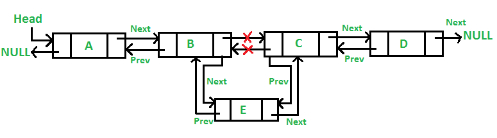/* Given a node as prev_node, insert a new node after the given node */
void insertAfter(struct node* prev_node, int new_data)
{
/*1. check if the given prev_node is NULL */
if (prev_node == NULL)
{
printf("the given previous node cannot be NULL");
return;
}

/* 2. allocate new node */
struct node* new_node =(struct node*) malloc(sizeof(struct node));

/* 3. put in the data  */
new_node->data  = new_data;

/* 4. Make next of new node as next of prev_node */
new_node->next = prev_node->next;

/* 5. Make the next of prev_node as new_node */
prev_node->next = new_node;

/* 6. Make prev_node as previous of new_node */
new_node->prev = prev_node;

/* 7. Change previous of new_node's next node */
if (new_node->next != NULL)
new_node->next->prev = new_node;
}

Five of the above steps step. The two extra steps are needed to change previous pointer of new node and previous pointer of new node’s next node.

3) Add a node at the end: (7 steps process)

The new node is always added after the last node of the given Linked List. For example if the given DLL is 5<->10<->15<->20<->25 and we add an item 30 at the end, then the DLL becomes 5<->10<->15<->20<->25<->30.
Since a Linked List is typically represented by the head of it, we have to traverse the list till end and then change the next of last node to new node.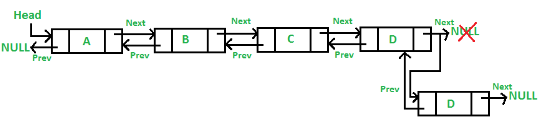Following are the 7 steps to add node at the end.
/* Given a reference (pointer to pointer) to the head
of a DLL and an int, appends a new node at the end  */
void append(struct node** head_ref, int new_data)
{
/* 1. allocate node */
struct node* new_node = (struct node*) malloc(sizeof(struct node));

struct node *last = *head_ref;  /* used in step 5*/

/* 2. put in the data  */
new_node->data  = new_data;

/* 3. This new node is going to be the last node, so
make next of it as NULL*/
new_node->next = NULL;

/* 4. If the Linked List is empty, then make the new
{
new_node->prev = NULL;
return;
}

/* 5. Else traverse till the last node */
while (last->next != NULL)
last = last->next;

/* 6. Change the next of last node */
last->next = new_node;

/* 7. Make last node as previous of new node */
new_node->prev = last;

return;
}

Six of the above 7 steps. The one extra step is needed to change previous pointer of new node.

4) Add a node before a given node

This is left as an exercise for the readers.

A complete working program to test above functions.

Following is complete C program to test above functions.

// A complete working C program to demonstrate all insertion methods
#include <stdio.h>
#include <stdlib.h>

struct node
{
int data;
struct node *next;
struct node *prev;
};

/* Given a reference (pointer to pointer) to the head of a list
and an int, inserts a new node on the front of the list. */
void push(struct node** head_ref, int new_data)
{
/* 1. allocate node */
struct node* new_node = (struct node*) malloc(sizeof(struct node));

/* 2. put in the data  */
new_node->data  = new_data;

/* 3. Make next of new node as head and previous as NULL */
new_node->prev = NULL;

/* 4. change prev of head node to new node */

/* 5. move the head to point to the new node */
}

/* Given a node as prev_node, insert a new node after the given node */
void insertAfter(struct node* prev_node, int new_data)
{
/*1. check if the given prev_node is NULL */
if (prev_node == NULL)
{
printf("the given previous node cannot be NULL");
return;
}

/* 2. allocate new node */
struct node* new_node =(struct node*) malloc(sizeof(struct node));

/* 3. put in the data  */
new_node->data  = new_data;

/* 4. Make next of new node as next of prev_node */
new_node->next = prev_node->next;

/* 5. Make the next of prev_node as new_node */
prev_node->next = new_node;

/* 6. Make prev_node as previous of new_node */
new_node->prev = prev_node;

/* 7. Change previous of new_node's next node */
if (new_node->next != NULL)
new_node->next->prev = new_node;
}

/* Given a reference (pointer to pointer) to the head
of a DLL and an int, appends a new node at the end  */
void append(struct node** head_ref, int new_data)
{
/* 1. allocate node */
struct node* new_node = (struct node*) malloc(sizeof(struct node));

struct node *last = *head_ref;  /* used in step 5*/

/* 2. put in the data  */
new_node->data  = new_data;

/* 3. This new node is going to be the last node, so
make next of it as NULL*/
new_node->next = NULL;

/* 4. If the Linked List is empty, then make the new
{
new_node->prev = NULL;
return;
}

/* 5. Else traverse till the last node */
while (last->next != NULL)
last = last->next;

/* 6. Change the next of last node */
last->next = new_node;

/* 7. Make last node as previous of new node */
new_node->prev = last;

return;
}

// This function prints contents of linked list starting from the given node
void printList(struct node *node)
{
struct node *last;
printf(" Traversal in forward direction ");
while (node != NULL)
{
printf(" %d ", node->data);
last = node;
node = node->next;
}

printf(" Traversal in reverse direction ");
while (last != NULL)
{
printf(" %d ", last->data);
last = last->prev;
}
}

/* Drier program to test above functions*/
int main()
{

// Insert 6.  So linked list becomes 6->NULL

// Insert 7 at the beginning. So linked list becomes 7->6->NULL

// Insert 1 at the beginning. So linked list becomes 1->7->6->NULL

// Insert 4 at the end. So linked list becomes 1->7->6->4->NULL

// Insert 8, after 7. So linked list becomes 1->7->8->6->4->NULL

printf("Created DLL is: ");

getchar();
return 0;
}

Output:

Created DLL is:
Traversal in forward direction
1  7  8  6  4
Traversal in reverse direction
4  6  8  7  1

Circular Linked List | (Introduction and Applications)

We have discussed singly and doubly linked lists in the following posts.

Introduction to linked List & Insertion
Doubly Linked List Introduction and Insertion

Circular linked list is a linked list where all nodes are connected to form a circle. There is no NULL at the end. A circular linked list can be a singly circular linked list or doubly circular linked list.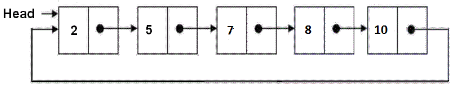1) Any node can be a starting point. We can traverse the whole list by starting from any point. We just need to stop when the first visited node is visited again.

2) Useful for implementation of queue. Unlike this implementation, we don’t need to maintain two pointers for front and rear if we use circular linked list. We can maintain a pointer to the last inserted node and front can always be obtained as next of last.

3) Circular lists are useful in applications to repeatedly go around the list. For example, when multiple applications are running on a PC, it is common for the operating system to put the running applications on a list and then to cycle through them, giving each of them a slice of time to execute, and then making them wait while the CPU is given to another application. It is convenient for the operating system to use a circular list so that when it reaches the end of the list it can cycle around to the front of the list.

4) Circular Doubly Linked Lists are used for implementation of advanced data structures.

The document Linked lists Notes | Study GATE Computer Science Engineering(CSE) 2022 Mock Test Series - Computer Science Engineering (CSE) is a part of the Computer Science Engineering (CSE) Course GATE Computer Science Engineering(CSE) 2022 Mock Test Series.
All you need of Computer Science Engineering (CSE) at this link: Computer Science Engineering (CSE)Use Code STAYHOME200 and get INR 200 additional OFF Use Coupon Code

Top Courses for Computer Science Engineering (CSE)GATE Computer Science Engineering(CSE) 2022 Mock Test Series

135 docs|165 tests

Top Courses for Computer Science Engineering (CSE)Track your progress, build streaks, highlight & save important lessons and more!

,

,

,

,

,

,

,

,

,

,

,

,

,

,

,

,

,

,

,

,

,

;